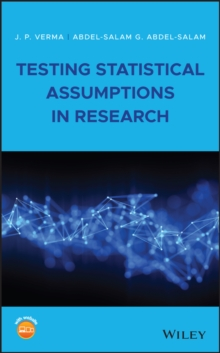Supporting your high street Find out how »
• My Account# Testing Statistical Assumptions in Research Hardback

#### Description

Comprehensively teaches the basics of testing statistical assumptions in research and the importance in doing so This book facilitates researchers in checking the assumptions of statistical tests used in their research by focusing on the importance of checking assumptions in using statistical methods, showing them how to check assumptions, and explaining what to do if assumptions are not met. Testing Statistical Assumptions in Research discusses the concepts of hypothesis testing and statistical errors in detail, as well as the concepts of power, sample size, and effect size.

It introduces SPSS functionality and shows how to segregate data, draw random samples, file split, and create variables automatically.

It then goes on to cover different assumptions required in survey studies, and the importance of designing surveys in reporting the efficient findings.

The book provides various parametric tests and the related assumptions and shows the procedures for testing these assumptions using SPSS software.

To motivate readers to use assumptions, it includes many situations where violation of assumptions affects the findings.

Assumptions required for different non-parametric tests such as Chi-square, Mann-Whitney, Kruskal Wallis, and Wilcoxon signed-rank test are also discussed.

Finally, it looks at assumptions in non-parametric correlations, such as bi-serial correlation, tetrachoric correlation, and phi coefficient. An excellent reference for graduate students and research scholars of any discipline in testing assumptions of statistical tests before using them in their research studyShows readers the adverse effect of violating the assumptions on findings by means of various illustrationsDescribes different assumptions associated with different statistical tests commonly used by research scholarsContains examples using SPSS, which helps facilitate readers to understand the procedure involved in testing assumptionsLooks at commonly used assumptions in statistical tests, such as z, t and F tests, ANOVA, correlation, and regression analysis Testing Statistical Assumptions in Research is a valuable resource for graduate students of any discipline who write thesis or dissertation for empirical studies in their course works, as well as for data analysts.

#### Information

• Format: Hardback
• Pages: 224 pages
• Publisher: John Wiley and Sons Ltd
• Publication Date:
• Category: Probability & statistics
• ISBN: 9781119528418

£87.50

£73.15We're closed for now, but hopefully not for long…

You can still add items to your basket and wishlist but you won't be able to checkout.